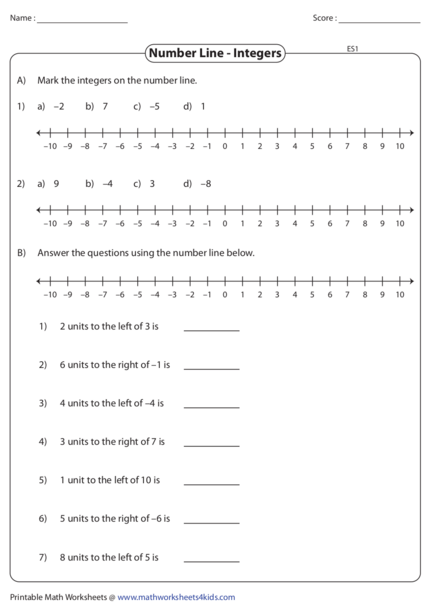# Worksheet on Number LineContributed by:Number Line worksheet. In elementary mathematics, a number line is a picture of a graduated straight line that serves as an abstraction for real numbers. Every point of a number line is assumed to correspond to a real number and every real number to a point.
1. Name : Score :
ES1
Number Line - Integers
A) Mark the integers on the number line.
1) a) –2 b) 7 c) –5 d) 1
–10 –9 –8 –7 –6 –5 –4 –3 –2 –1 0 1 2 3 4 5 6 7 8 9 10
2) a) 9 b) –4 c) 3 d) –8
–10 –9 –8 –7 –6 –5 –4 –3 –2 –1 0 1 2 3 4 5 6 7 8 9 10
B) Answer the questions using the number line below.
–10 –9 –8 –7 –6 –5 –4 –3 –2 –1 0 1 2 3 4 5 6 7 8 9 10
1) 2 units to the left of 3 is
2) 6 units to the right of –1 is
3) 4 units to the left of –4 is
4) 3 units to the right of 7 is
5) 1 unit to the left of 10 is
6) 5 units to the right of –6 is
7) 8 units to the left of 5 is
Printable Math Worksheets @ www.mathworksheets4kids.com
2. Name : Score :
ES1
Number Line - Integers
A) Mark the integers on the number line.
1) a) –2 b) 7 c) –5 d) 1
–10 –9 –8 –7 –6 –5 –4 –3 –2 –1 0 1 2 3 4 5 6 7 8 9 10
2) a) 9 b) –4 c) 3 d) –8
–10 –9 –8 –7 –6 –5 –4 –3 –2 –1 0 1 2 3 4 5 6 7 8 9 10
B) Answer the questions using the number line below.
–10 –9 –8 –7 –6 –5 –4 –3 –2 –1 0 1 2 3 4 5 6 7 8 9 10
1) 2 units to the left of 3 is 1
2) 6 units to the right of –1 is 5
3) 4 units to the left of –4 is –8
4) 3 units to the right of 7 is 10
5) 1 unit to the left of 10 is 9
6) 5 units to the right of –6 is –1
7) 8 units to the left of 5 is –3
Printable Math Worksheets @ www.mathworksheets4kids.com